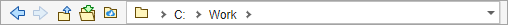## MATLAB 可访问的文件和文件夹

### MATLAB 在什么位置查找文件？

• 将当前文件夹更改为包含这些文件的文件夹。您可以使用 `cd` 函数，也可以使用当前文件夹工具栏浏览到其他文件夹：• 将包含文件的文件夹添加至搜索路径。您对搜索路径的更改将应用于当前的 MATLAB 会话。要在以后的 MATLAB 会话中重新使用修改后的搜索路径，可以保存您所做的更改。

• `userpath MATLAB` 文件夹中存储个别文件，该文件夹位于搜索路径上。要确定该文件夹的位置，请运行 `userpath` 函数。

### 您应添加至搜索路径的文件和文件夹

MATLAB 搜索路径应包括：

• 包含您运行的文件的文件夹。

• 包含由您运行的文件调用的文件的文件夹。

• 包含您运行的文件的子文件夹。使文件夹成为可访问的文件夹并不能使其子文件夹成为可访问的文件夹。

### 当多个文件同名时

MATLAB 使用的文件称为遮蔽文件。在某些情况下，MATLAB 会提示您存在遮蔽文件。

### MathWorks 产品的位置

MathWorks 提供的产品的文件和文件夹位于 `matlabroot``/toolbox` 中。`matlabroot` 下的文件和文件夹对您的安装很重要。尤其是：

• 要将您的个人文件和文件夹存储在 `matlabroot``/toolbox` 中。

• 要更改 `matlabroot``/toolbox` 中的文件、文件夹和子文件夹。除了 `pathdef.m` 文件，您可以更新该文件并将它保存在自己的默认位置 `matlabroot``/toolbox/local`

`dir(fullfile(matlabroot,'toolbox'))`